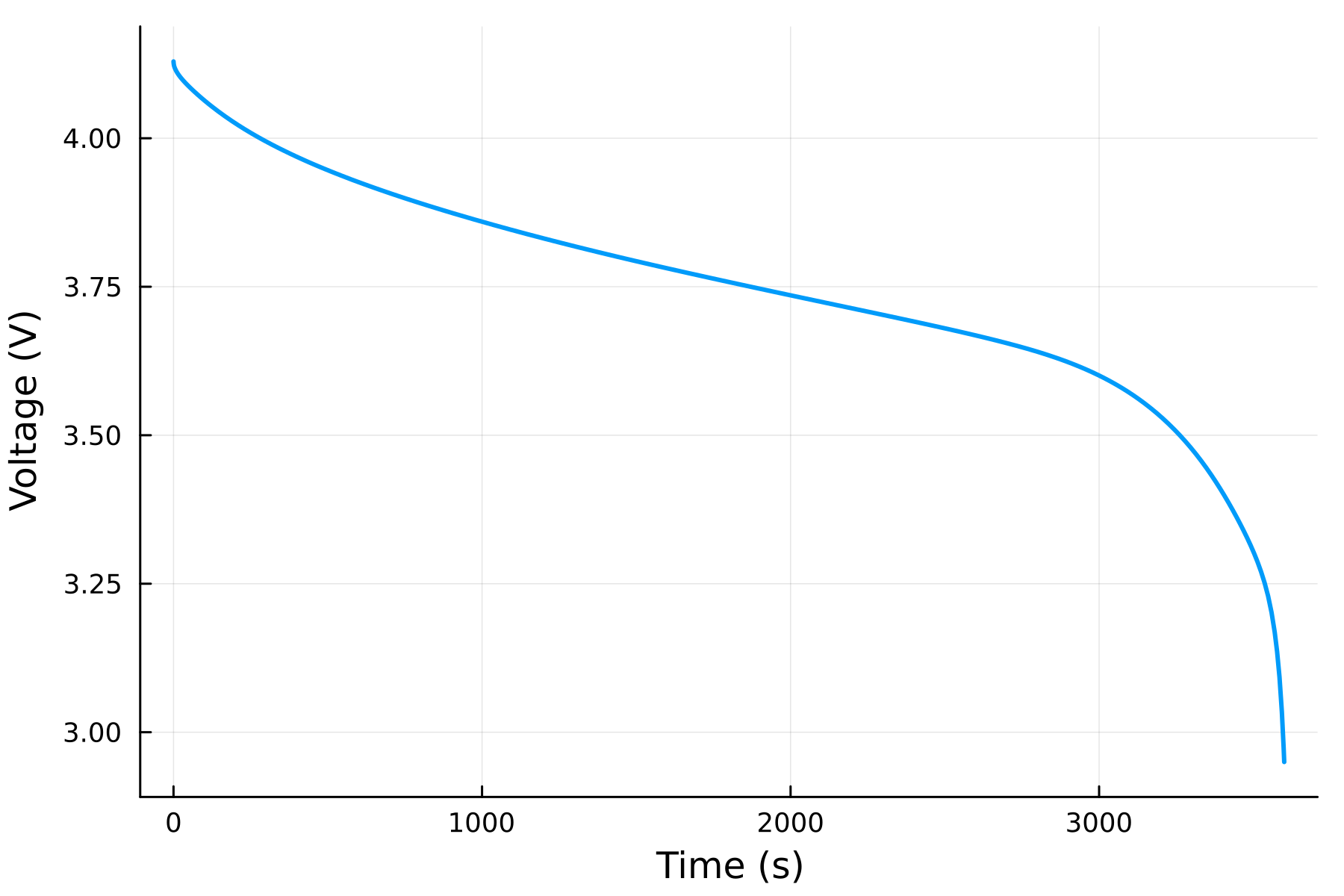## Simple discharge experiment

To run a simple experiment on the cell, we can use the solve function. The first argument is cell created above, and the second argument is the type of experiment we want to run. Here, we run a discharge experiment at a C-rate of 1C. C-rate is a measure of the current relative to the capacity of the battery, where 1C fully discharges a battery in 60 minutes and a C-rate of 2C fully discharges a battery in 30 minutes. A default initial state of charge (SOC) is given in the function for the LCO chemistry, and we can override that by passing in a value for SOC_initial.

sol = solve(cell, discharge(1C); SOC_initial = 1)

The output of the simulation sol contains the time series of the states of the battery. We can plot the voltage of the battery using the plot function. We can access the states by indexing sol, such as

sol["voltage"]

The available states can be accessed by evaluating getstates on the cell

getstates(cell)

and plotted using the Plots package

using Plots
plot(sol, "voltage")